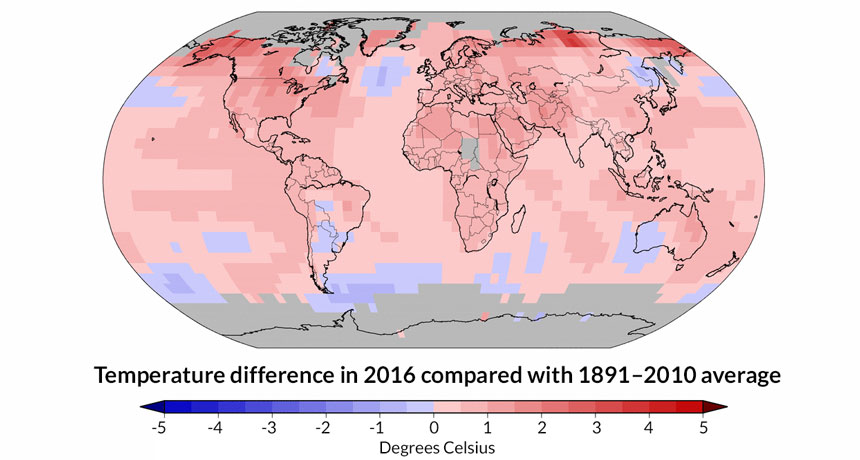We saw that, depending on the parameter, the Mandelbrot law can either "explode" when iterated or reach a steady state. It would be interesting to see for which parameters an "explosion" (a.k.a. a "divergence") happens. However, the parameter can be a complex number, so we need two dimensions to describe all possible parameters (e.g. x axis for the real component of the number and y axis for the imaginary component of the number). Then in color we can mark whether (or when) the iterations of the Mandelbrot law diverge. Such a type of 2D maps is called "heat maps", similar to the ones used in weather forecasts.Side note: The colors attributed to different values are arbitrary. Above we picked blue for cold and red for hot, but we could have picked anything we want. The particular choice is called "the color map" or "the color scheme" of the plot (it has nothing to do with geographical maps). Later today we will see how to use custom color maps.

Below we give you the code that can be used to plot the heat map of "divergence of the Mandelbrot law versus parameter used in its evaluation". Let us play with it before trying to explain exactly how it works.

In :
using Plots

function mandelbrot_law(current_state, parameter)
next_state = current_state^2+parameter
return next_state
end

function mandelbrot_when_explodes(parameter, length)
current_state = 0
for i in 2:length
new_state = mandelbrot_law(current_state, parameter)
if abs(new_state) > 20
return i
else
current_state = new_state
end
end
return length
end

function get_grid_of_coordinates(rmin,rmax,imin,imax,step)
real_coordinate = rmin:step:rmax
imag_coordinate = imin:step:imax
grid_of_complex_coordinates = zeros(ComplexF64, length(imag_coordinate), length(real_coordinate))

for i_real in 1:length(real_coordinate)
for i_imag in 1:length(imag_coordinate)
grid_of_complex_coordinates[i_imag,i_real] = real_coordinate[i_real] + 1im * imag_coordinate[i_imag]
end
end
return grid_of_complex_coordinates
end

function plot_mandelbrot(rmin,rmax,imin,imax,step,length)
grid_of_complex_coordinates = get_grid_of_coordinates(rmin,rmax,imin,imax,step)
heatmap(rmin:step:rmax,imin:step:imax, mandelbrot_when_explodes.(grid_of_complex_coordinates, length), ratio=1)
end